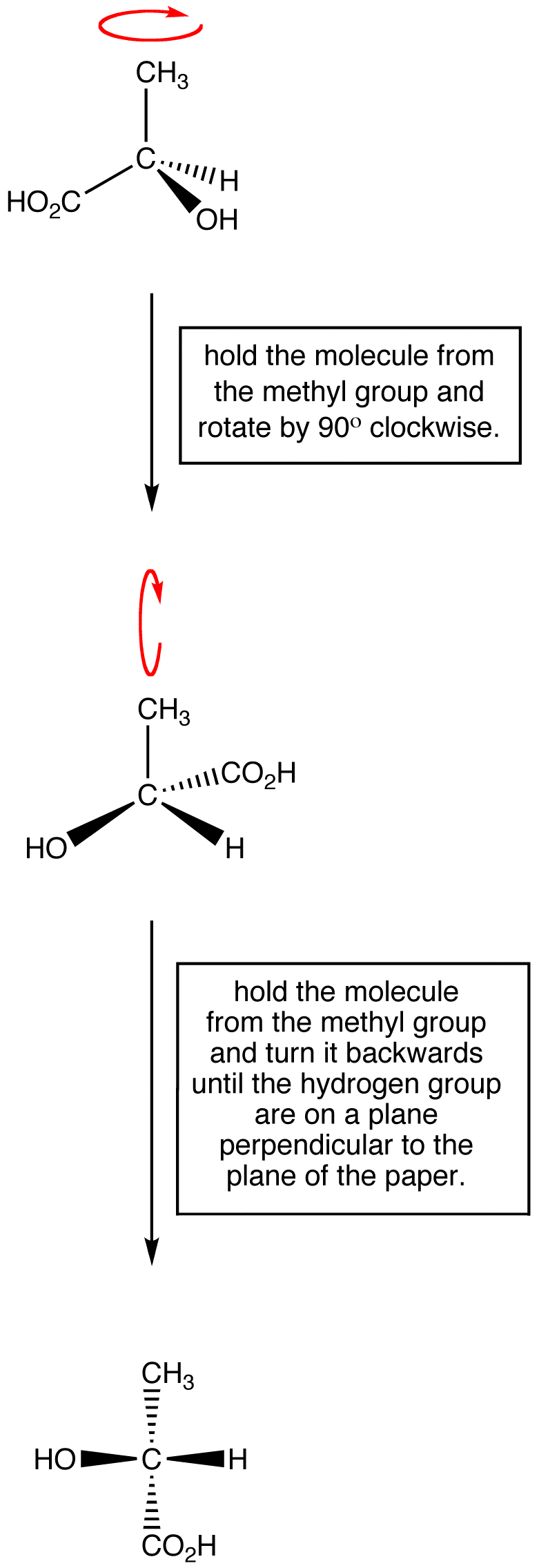# 2.3.2: Fischer Projections to communicate Chirality

$$\newcommand{\vecs}{\overset { \rightharpoonup} {\mathbf{#1}} }$$ $$\newcommand{\vecd}{\overset{-\!-\!\rightharpoonup}{\vphantom{a}\smash {#1}}}$$$$\newcommand{\id}{\mathrm{id}}$$ $$\newcommand{\Span}{\mathrm{span}}$$ $$\newcommand{\kernel}{\mathrm{null}\,}$$ $$\newcommand{\range}{\mathrm{range}\,}$$ $$\newcommand{\RealPart}{\mathrm{Re}}$$ $$\newcommand{\ImaginaryPart}{\mathrm{Im}}$$ $$\newcommand{\Argument}{\mathrm{Arg}}$$ $$\newcommand{\norm}{\| #1 \|}$$ $$\newcommand{\inner}{\langle #1, #2 \rangle}$$ $$\newcommand{\Span}{\mathrm{span}}$$ $$\newcommand{\id}{\mathrm{id}}$$ $$\newcommand{\Span}{\mathrm{span}}$$ $$\newcommand{\kernel}{\mathrm{null}\,}$$ $$\newcommand{\range}{\mathrm{range}\,}$$ $$\newcommand{\RealPart}{\mathrm{Re}}$$ $$\newcommand{\ImaginaryPart}{\mathrm{Im}}$$ $$\newcommand{\Argument}{\mathrm{Arg}}$$ $$\newcommand{\norm}{\| #1 \|}$$ $$\newcommand{\inner}{\langle #1, #2 \rangle}$$ $$\newcommand{\Span}{\mathrm{span}}$$

Learning Objective

• draw, interpret, and convert between perspective formulae and Fischer projections for chiral compounds

## Perspective Formulas and Fischer Projections

So far, we have communicated the stereochemical orentation of compounds using the wedges and dashes of perspective formulas. For example, the perspective formula for (R)-Lactic acid is shown below.A Fischer projection is a convention used to depict stereochemistry in two dimensions. The horizontal bonds are seen as wedges and the vertical bonds are seen as dashed lines as shown below in the example below for glyceraldehyde.## Converting between Perspective and Fischer Formulas

It can be useful to convert between perspective formulas and Fischer projections. Below is one approach using (R)-lactic acid as an example..

Step 1: Hold the molecule so that

a) the chiral center is on the plane of the paper,

b) two bonds are coming out of the plane of the paper and are on a horizontal plane,

c) the two remaining bonds are going into the plane of the paper and are on a vertical planeStep 2: Push the two bonds coming out of the plane of the paper onto the plane of the paper.Step 3: Pull the two bonds going into the plane of the paper onto the plane of the paper.Step 4: Omit the chiral atom symbol for convenience. This is the Fischer Projection of (R)-Lactic acid.The stereochemical formula for (R)-lactic acid can be drawn using either method. To build this skill, we begin by drawing the structures and converting them step wise. Models can also be helpful. Eventually, we will be able to mentally conversion between these two structures.Exercise 1

1. Convert each compound into the alternate sterochemical structure (PerspectiveFischer).